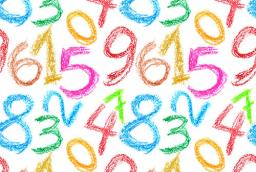# Larger) 3381

Which number a is 15 smaller (b - larger) than its half?

a =  -30
b =  30

### Step-by-step explanation:

a=a/2-15

a = -30

a = -30/1 = -30

a = -30

Our simple equation calculator calculates it.

b=b/2+15

b = 30

b = 30/1 = 30

b = 30

Our simple equation calculator calculates it.Did you find an error or inaccuracy? Feel free to write us. Thank you!

Tips for related online calculators
Do you have a linear equation or system of equations and looking for its solution? Or do you have a quadratic equation?